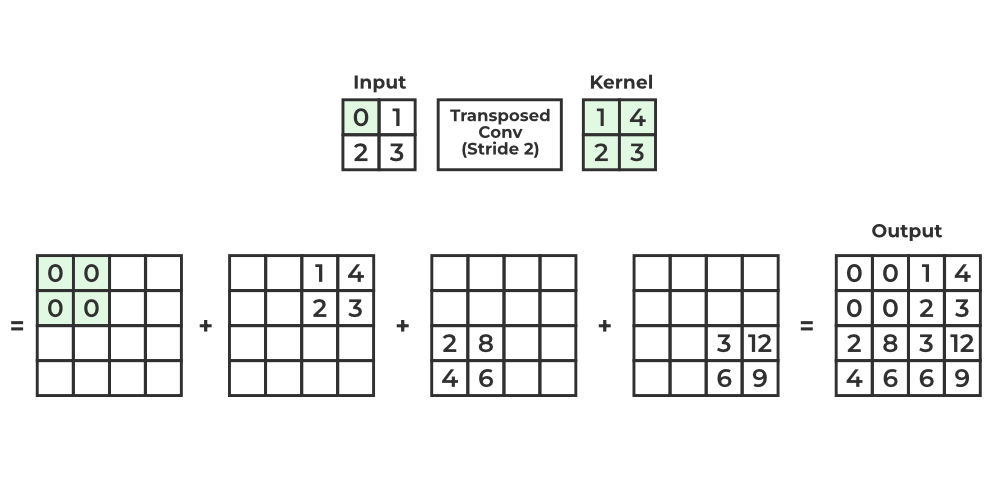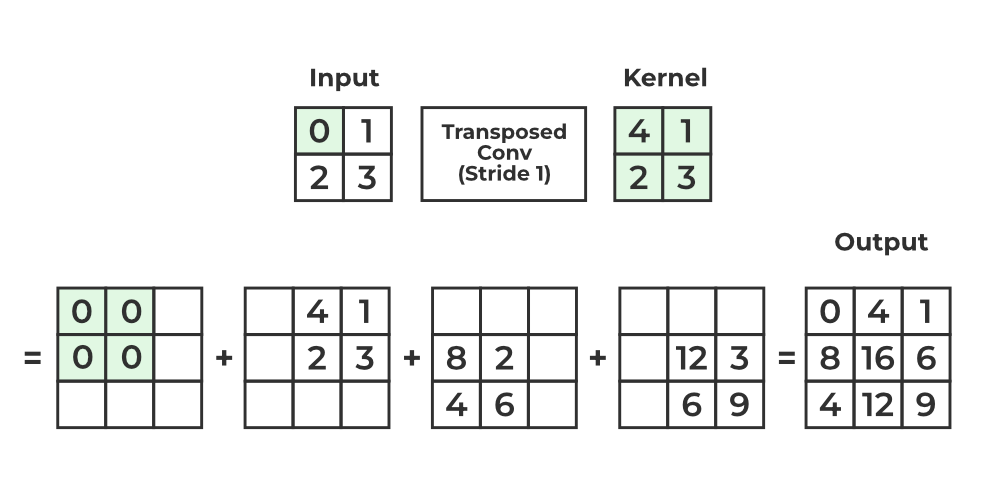Open in App
Not now

# What is Transposed Convolutional Layer?

• Last Updated : 24 Jan, 2023

A transposed convolutional layer is an upsampling layer that generates the output feature map greater than the input feature map. It is similar to a deconvolutional layer. A deconvolutional layer reverses the layer to a standard convolutional layer. If the output of the standard convolution layer is deconvolved with the deconvolutional layer then the output will be the same as the original value, While in transposed convolutional value will not be the same, it can reverse to the same dimension,

Transposed convolutional layers are used in a variety of tasks, including image generation, image super-resolution, and image segmentation. They are particularly useful for tasks that involve upsampling the input data, such as converting a low-resolution image to a high-resolution one or generating an image from a set of noise vectors.

The operation of a transposed convolutional layer is similar to that of a normal convolutional layer, except that it performs the convolution operation in the opposite direction. Instead of sliding the kernel over the input and performing element-wise multiplication and summation, a transposed convolutional layer slides the input over the kernel and performs element-wise multiplication and summation. This results in an output that is larger than the input, and the size of the output can be controlled by the stride and padding parameters of the layer.Transposed Convolutional  with stride 2

In a transposed convolutional layer, the input is a feature map of size, whereandare the height and width of the input and the kernel size is, whereandare the height and width of the kernel.

If the stride shape isand the padding is p, The stride of the transposed convolutional layer determines the step size for the input indices p and q, and the padding determines the number of pixels to add to the edges of the input before performing the convolution. Then the output of the transposed convolutional layer will bewhereandare the height and width of the output.

### Example 1:

Suppose we have a grayscale image of size 2 X 2, and we want to upsample it using a transposed convolutional layer with a kernel size of 2 x 2, a stride of 1, and zero padding (or no padding). The input image and the kernel for the transposed convolutional layer would be as follows:The output will be:Transposed Convolutional  Stride = 1

#### Method 1: Manually with TensorFlow

Code Explanations:

• Import necessary libraries (TensorFlow and NumPy)
• Define Input tensor and custom kernel
• Apply Transpose convolution with kernel size =2, stride = 1.
• Write the custom functions for transpose convolution
• Apply Transpose convolution on input data.

## Python3

 # Import Tensorflow import tensorflow as tf import numpy as np   # Input Input = tf.constant([[0.0, 1.0], [2.0, 3.0]], dtype=tf.float32) # Kernel Kernel = tf.constant([[4.0, 1.0], [2.0, 3.0]], dtype=tf.float32) # Define the transpose convolution function     def trans_conv(Input, Kernel):     h, w = Kernel.shape     Y = np.zeros((Input.shape + h - 1, Input.shape + w - 1))       for i in range(Input.shape):         for j in range(Input.shape):             Y[i: i + h, j: j + w] += Input[i, j] * Kernel     return tf.constant(Y)     # OUTPUT trans_conv(Input, Kernel)

Output:

<tf.Tensor: shape=(3, 3), dtype=float64, numpy=
array([[ 0.,  4.,  1.],
[ 8., 16.,  6.],
[ 4., 12.,  9.]])>

The output shape can be calculated as :#### Method 2: With PyTorch:

Code Explanations:

• Import necessary libraries (torch and nn from torch)
• Define Input tensor and custom kernel
• Redefine the shape in 4 dimensions because PyTorch takes 4D shapes in inputs.
• Apply Transpose convolution with input and output channel =1,1, kernel size =2, stride = 1, padding = 0 means valid padding.
• Set the customer kernel weight by using Transpose.weight.data
• Apply Transpose convolution on input data.

## Python3

 # Import the necessary module import torch from torch import nn   # Input Input = torch.tensor([[0.0, 1.0], [2.0, 3.0]]) #Kernel Kernel = torch.tensor([[4.0, 1.0], [2.0, 3.0]])   # Redefine the shape in 4 dimension Input = Input.reshape(1, 1, 2, 2) Kernel = Kernel.reshape(1, 1, 2, 2)   # Transpose convolution Layer Transpose = nn.ConvTranspose2d(in_channels =1,                                 out_channels =1,                                kernel_size=2,                                 stride = 1,                                 padding=0,                                 bias=False)   # Initialize Kernel Transpose.weight.data = Kernel # Output value Transpose(Input)

Output:

tensor([[[[ 0.,  4.,  1.],
[ 8., 16.,  6.],
[ 4., 12.,  9.]]]], grad_fn=<ConvolutionBackward0>)

Transposed convolutional layers are often used in conjunction with other types of layers, such as pooling layers and fully connected layers, to build deep convolutional networks for various tasks.

## Python3

 import tensorflow as tf   # Define the input tensor input_tensor = tf.constant([[     [[1, 2, 3], [5, 6, 7], [9, 10, 11], [13, 14, 15]],     [[17, 18, 19], [21, 22, 23], [25, 26, 27], [29, 30, 31]],     [[33, 34, 35], [37, 38, 39], [41, 42, 43], [45, 46, 47]],     [[49, 50, 51], [53, 54, 55], [57, 58, 59], [61, 62, 63]] ]], dtype=tf.float32)     # Define the transposed convolutional layer transposed_conv_layer = tf.keras.layers.Conv2DTranspose(     filters=1, kernel_size=3, strides=2, padding='valid')   # Apply the transposed convolutional layer to the input tensor output = transposed_conv_layer(input_tensor)   # Print the output shape print(output.shape)

Output:

(1, 9, 9, 1)

## Python3

 import tensorflow as tf   # Define the input tensor input_tensor = tf.constant([[     [[1, 2, 3], [5, 6, 7], [9, 10, 11], [13, 14, 15]],     [[17, 18, 19], [21, 22, 23], [25, 26, 27], [29, 30, 31]],     [[33, 34, 35], [37, 38, 39], [41, 42, 43], [45, 46, 47]],     [[49, 50, 51], [53, 54, 55], [57, 58, 59], [61, 62, 63]] ]], dtype=tf.float32)     # Define the transposed convolutional layer transposed_conv_layer = tf.keras.layers.Conv2DTranspose(     filters=1, kernel_size=3, strides=2, padding='same')   # Apply the transposed convolutional layer to the input tensor output = transposed_conv_layer(input_tensor)   # Print the output shape print(output.shape)

Output:

(1, 8, 8, 1)

My Personal Notes arrow_drop_up
Related Articles